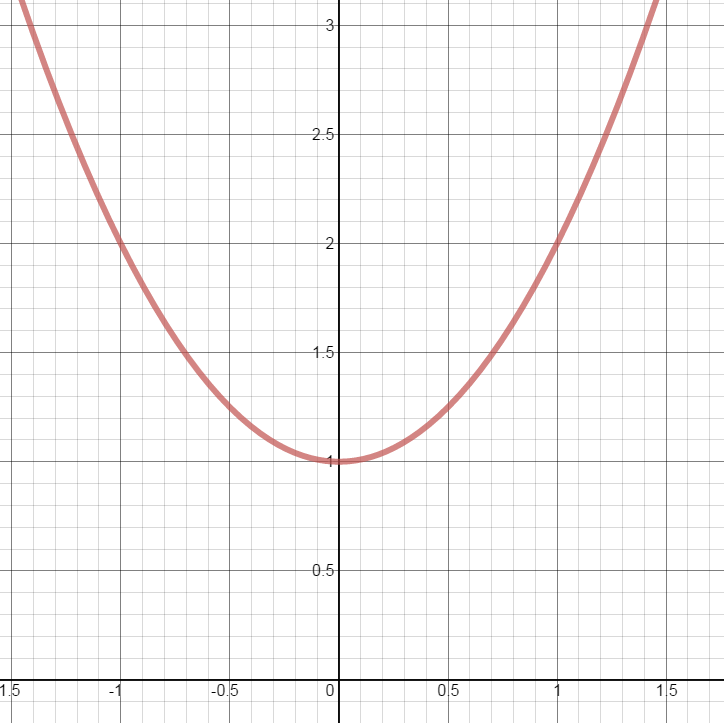### Day 71 - Evaluating Functions - 12.03.14

• Functions Quiz tomorrow!

Bell Ringer
• Functions
1. What is a function?

1. a mathematical relationship in which any output has one and only one input

2. a mathematical relationship in which any input has one and only one output

3. a mathematical relationship in which any input has at least one output

4. a mathematical relationship in which any output has at least one input

5. none of the above

2. What is the domain and range of the following function? Make window full screen to enlarge image.1. Domain: x > 1 | Range: all real numbers

2. Domain: x < 1 | Range: all real numbers

3. Domain: all real numbers | Range: all real numbers

4. Domain: all real numbers | Range: y > 1

5. none of the above

1. 0

2. 1

3. -1

4. 5

5. none of the above

Review
• Functions
• Domain
• Range
• Evaluating Functions

Lesson

Exit Ticket
• Posted on board at end of block.
Lesson Objective(s)
• Function Work Day!

#### In-Class Help Requests

Standard(s)
• N/A

Mathematical Practice(s)
• #1 - Make sense of problems and persevere in solving them
• #2 - Reason abstractly and quantitatively
• #4 - Model with mathematics
• #5 - Use appropriate tools strategically
• #7 - Look for and make use of structure

Past Checkpoints
• N/A